Untitled

### Introduction¶

A somewhat neglected model in the Data Science/Machine Learning community are Multivariate Adaptive Regression Splines (MARS). Despite being less popular than Neural Networks or Trees, I find the concept of MARS pretty interesting, especially because it comes as a fully linear model and thus can preserve model interpretability.

One of the drawbacks of the standard MARS algorithm is the greedy selection scheme for basis functions. Like in CART based algorithms, MARS only looks for the locally best term to add or remove. While this still leads to good results in many cases, it would be cool to also find a globally optimal solution.

This is actually quite simple with Penalized Regression, although the computational complexity grows quickly with data-size.

### The data¶

The major increase in complexity for this approach comes from taking higher order interactions into account. For that reason, I’ll keep the data pretty simple for now.

The function that I have (arbitrarily) decided to use for demonstration is

$$f(x) = sin(x)+\frac{1}{2}x+\epsilon,\quad x\in[-5,5].$$

As a training set, I drew 1000 samples uniformly from $[-5,5]$, applied the function and added Gaussian $\mathcal{N}(0;0.3)$ noise. Thus, the sampled data follows

$$y = sin(x)+\frac{1}{2}x+\epsilon,\quad \epsilon\sim\mathcal{N}(0;0.3),\quad x\sim\mathcal{Unif}(-5,5)$$

The test set simply consists of another 1000 points scattered evenly in $[-5,5]$.

In :
import pandas as pd
import numpy as np

from sklearn.linear_model import Ridge, Lasso

import matplotlib.pyplot as plt

from mpl_toolkits.mplot3d import Axes3D
import matplotlib.pyplot as plt
from matplotlib import cm

In :
np.random.seed(123)
x = pd.Series(np.random.uniform(-5,5,1000))
y = pd.Series(np.sin(x) + 0.5*x +  np.random.normal(loc = 0, scale = .3, size = 1000))

#The grid is used to plot the actual noise-less function that we want to approximate
x_grid = pd.Series(np.linspace(-5,5,1000))
y_grid = pd.Series(np.sin(x_grid) + 0.5*x_grid)

In :
plt.figure(figsize = (8,6))
plt.scatter(x,y)
plt.plot(x_grid, y_grid, color = "orange", lw = 4)

plt.legend(["Truth", "Random Draws"])

Out:
<matplotlib.legend.Legend at 0x7f166779eba8>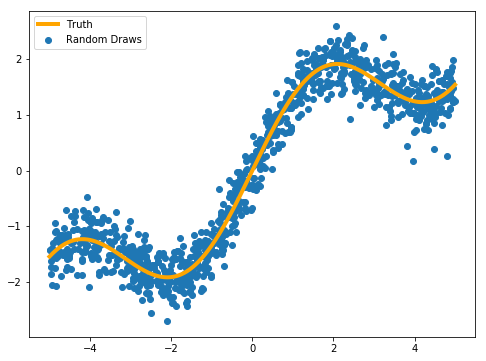### The model¶

For the univariate case, we want to use every training example as a possible knot point for the spline and let the penalized regression find the optimal linear combination among all possible knots.

The model in question is therefore

$$\hat{f}(x) = \beta_0 + \sum_{i=1}^N \beta_i\cdot max(0,x – x_i)$$

where $N$ is the size of the training data. I chose Lasso here to make the result look more like Piecewiese Linear Regression. In MARS, the reverse function $max(0,x_i – x)$ would actually also be used as a regressor. To avoid explosion of features, I left these mirrored hinge functions out, recognizing no disadvantages in results.

Now, we start by creating the feature matrices. As a test set, I used 1000 evenly spaced points on $[-5,5]$ to plot the resulting regression function (see above).

In :
mars_matrix_train = np.zeros((1000,1000))
mars_matrix_test = np.zeros((1000,1000))

for row in range(1000):
mars_matrix_train[:,row] = x.apply(lambda a: np.maximum(0.,a-x.iloc[row])).values
mars_matrix_test[:,row] = x_grid.apply(lambda a: np.maximum(0.,a-x.iloc[row])).values


The $\alpha$ (penalization) parameter should actually be selected via some sort of cross validation to find its optimimum given the data. For this toy example, I just tested a little bit until I found a feasible solution.

In :
lasso = Lasso(.01)
lasso.fit(mars_matrix_train, y.values.reshape(-1,1))

y_pred = lasso.predict(mars_matrix_test)

plt.figure(figsize = (8,6))
plt.scatter(x,y)
plt.plot(x_grid, y_grid, color = "orange", lw = 4)

plt.plot(x_grid,y_pred, color = "red", lw=3)
plt.legend(["Truth", "Predicted", "Random Draws"])

/usr/local/lib/python3.6/dist-packages/sklearn/linear_model/coordinate_descent.py:491: ConvergenceWarning: Objective did not converge. You might want to increase the number of iterations. Fitting data with very small alpha may cause precision problems.
ConvergenceWarning)

Out:
<matplotlib.legend.Legend at 0x7f16675f5860>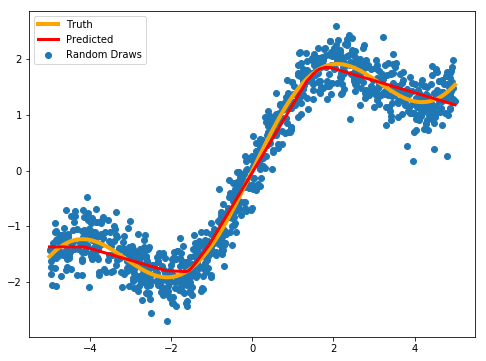By decreasing $\alpha$, the function becomes smoother. For small data-sizes, it is reasonable to keep the penalization higher to avoid overfit. In this case, decreasing the penalization parameter actually improved accuracy:

In :
lasso = Lasso(.001)
lasso.fit(mars_matrix_train, y.values.reshape(-1,1))

y_pred = lasso.predict(mars_matrix_test)

plt.figure(figsize = (8,6))
plt.scatter(x,y)
plt.plot(x_grid, y_grid, color = "orange", lw = 4)

plt.plot(x_grid,y_pred, color = "red", lw=3)
plt.legend(["Truth", "Predicted", "Random Draws"])

/usr/local/lib/python3.6/dist-packages/sklearn/linear_model/coordinate_descent.py:491: ConvergenceWarning: Objective did not converge. You might want to increase the number of iterations. Fitting data with very small alpha may cause precision problems.
ConvergenceWarning)

Out:
<matplotlib.legend.Legend at 0x7f1667539a20>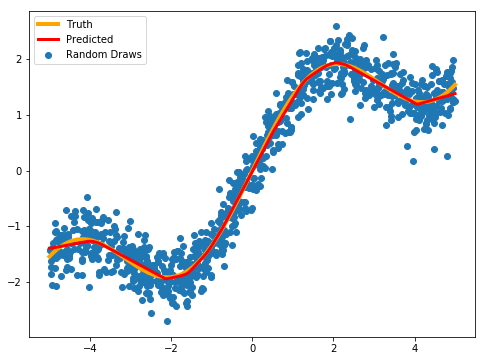To get even more smoothness, Ridge Regression could be used instead of Lasso.

In :
lasso = Ridge()
lasso.fit(mars_matrix_train, y.values.reshape(-1,1))

y_pred = lasso.predict(mars_matrix_test)

plt.figure(figsize = (8,6))
plt.scatter(x,y)
plt.plot(x_grid, y_grid, color = "orange", lw = 4)

plt.plot(x_grid,y_pred, color = "red", lw=3)
plt.legend(["Truth", "Predicted", "Random Draws"])

Out:
<matplotlib.legend.Legend at 0x7f166751cdd8>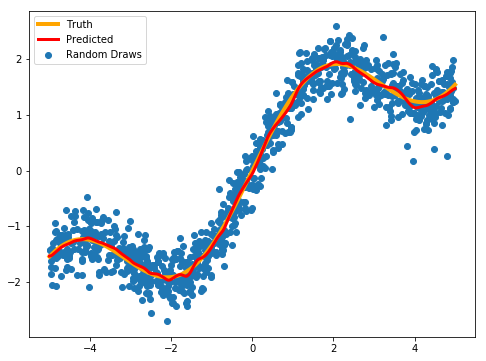However, Ridge Regression tended to be more prone to overfit and we also don’t get the kind of variable selection that we do get from Lasso:

In :
lasso = Ridge(.1)
lasso.fit(mars_matrix_train, y.values.reshape(-1,1))

y_pred = lasso.predict(mars_matrix_test)

plt.figure(figsize = (8,6))
plt.scatter(x,y)
plt.plot(x_grid, y_grid, color = "orange", lw = 4)

plt.plot(x_grid,y_pred, color = "red", lw=3)
plt.legend(["Truth", "Predicted", "Random Draws"])

Out:
<matplotlib.legend.Legend at 0x7f166747fd68>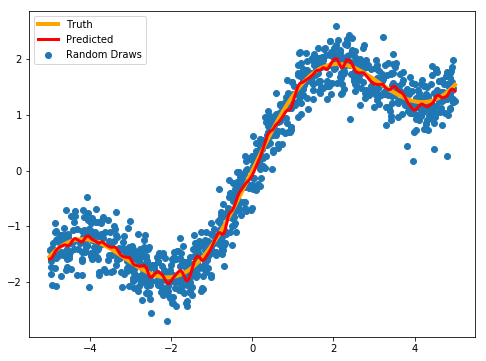### Dealing with higher dimensionality¶

The next generalizing step takes us to higher dimensional regression problems. In order to be able to visualize, I will stick with the 2-dimensional case. The function for demonstration the 2D case is

$$f(x,y)= sin(2x)+\frac{1}{2}x+cos(2y)-\frac{1}{2}y,\quad x,y\in[-1,1]$$

For training set, I sampled again independently uniform on the domains of $x$ and $y$, applied $f(x,y)$ and added the same Gaussian $\mathcal{N}(0;0.3)$ noise as before:

$$z = sin(2x)+\frac{1}{2}x+cos(2y)-\frac{1}{2}y,\quad \epsilon\sim\mathcal{N}(0;0.3),\quad x,y\sim\mathcal{Unif}(-1,1)$$

The reason why I reduced the interval for $x$ and $y$ was to reduce the additional spacing among datapoints that comes with the adding one dimension (curse of dimensionality).

In :
np.random.seed(123)

#only 50 points per dimension, as the meshgrid will eventually make it 50 X 50 = 2,500 points
X_coordinates = np.linspace(-1,1,50).reshape(-1,1)
Y_coordinates = np.linspace(-1,1,50).reshape(-1,1)

xx_grid, yy_grid = np.meshgrid(X_coordinates, Y_coordinates)

point_space = np.c_[xx_grid.ravel(), yy_grid.ravel()]

Z_coordinates_truth = pd.Series(np.sin(point_space[:,0]*2) + 0.5*point_space[:,0] +\
np.cos(point_space[:,1]*2) - 0.5*point_space[:,1])

zz_grid_truth = Z_coordinates_truth.values.reshape(50,50)

X_coordinates_sample = np.random.uniform(-1,1,1000)
Y_coordinates_sample = np.random.uniform(-1,1,1000)

Z_coordinates_sample = pd.Series(np.sin(X_coordinates_sample*2) + 0.5*X_coordinates_sample +\
np.cos(Y_coordinates_sample*2) - 0.5*Y_coordinates_sample) +\
np.random.normal(0,.3, 1000)

x = pd.Series(X_coordinates_sample)
y = pd.Series(Y_coordinates_sample)

x_test = pd.Series(point_space[:,0])
y_test = pd.Series(point_space[:,1])

fig = plt.figure(figsize = (12,12))
ax = fig.gca(projection='3d')
ax.plot_surface(xx_grid, yy_grid, zz_grid_truth, cmap=cm.coolwarm,
linewidth=0, antialiased=False)

Out:
<mpl_toolkits.mplot3d.art3d.Poly3DCollection at 0x7f16671e4e48>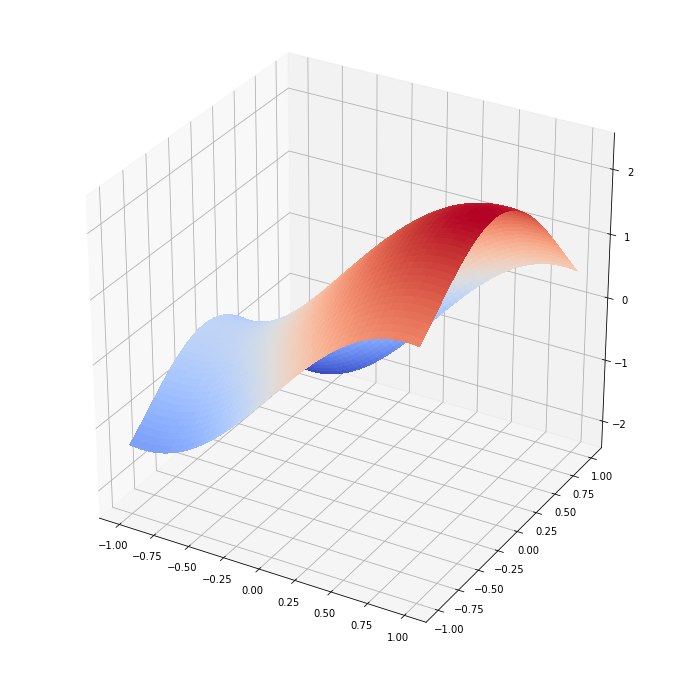To account for the additional dimension, we need to double the amount of regressors (excluding the constant) in order to stay consistent with the 1D framework – the 2D model is then:

$$\hat{f}(x,y) = \beta_0 + \sum_{i=1}^N \beta_{xi}\cdot max(0,x – x_{i})+\beta_{yi}\cdot max(0,y – y_{i})$$

And we can put that into code:

In :
mars_matrix_train = np.zeros((1000,2000))

mars_matrix_test = np.zeros((2500,2000))

#x
for row in range(1000):
mars_matrix_train[:,row] = x.apply(lambda a: np.maximum(0.,a-x.iloc[row])).values
mars_matrix_test[:,row] = x_test.apply(lambda a: np.maximum(0.,a-x.iloc[row])).values
#y
for row in range(1000):
mars_matrix_train[:,1000+row] = y.apply(lambda a: np.maximum(0.,a-y.iloc[row])).values
mars_matrix_test[:,1000+row] = y_test.apply(lambda a: np.maximum(0.,a-y.iloc[row])).values


Now, the same principles as before apply. Higher penalization leads to a less smooth solution and vice-versa (I left out Ridge for the 2D case):

In :
lasso = Lasso(.01)
lasso.fit(mars_matrix_train, Z_coordinates_sample.values.reshape(-1,1))

z_pred = lasso.predict(mars_matrix_test).reshape(50,50)

fig = plt.figure(figsize = (16,12))

ax = fig.add_subplot(1, 2, 1, projection='3d')
ax.plot_surface(xx_grid, yy_grid, z_pred, cmap=cm.coolwarm,
linewidth=0, antialiased=False)
plt.title("Estimated")

ax = fig.add_subplot(1, 2, 2, projection='3d')
ax.plot_surface(xx_grid, yy_grid, zz_grid_truth, cmap=cm.coolwarm,
linewidth=0, antialiased=False)
plt.title("Truth")

Out:
Text(0.5,0.92,'Truth')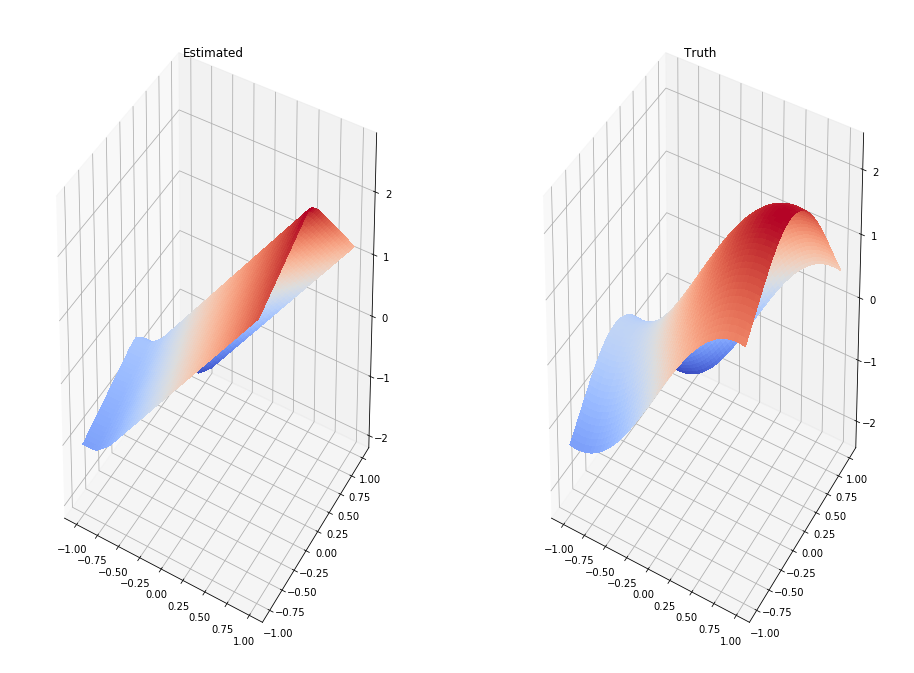In :
lasso = Lasso(.001)
lasso.fit(mars_matrix_train, Z_coordinates_sample.values.reshape(-1,1))

z_pred = lasso.predict(mars_matrix_test).reshape(50,50)

fig = plt.figure(figsize = (16,12))

ax = fig.add_subplot(1, 2, 1, projection='3d')
ax.plot_surface(xx_grid, yy_grid, z_pred, cmap=cm.coolwarm,
linewidth=0, antialiased=False)
plt.title("Estimated")

ax = fig.add_subplot(1, 2, 2, projection='3d')
ax.plot_surface(xx_grid, yy_grid, zz_grid_truth, cmap=cm.coolwarm,
linewidth=0, antialiased=False)
plt.title("Truth")

/usr/local/lib/python3.6/dist-packages/sklearn/linear_model/coordinate_descent.py:491: ConvergenceWarning: Objective did not converge. You might want to increase the number of iterations. Fitting data with very small alpha may cause precision problems.
ConvergenceWarning)

Out:
Text(0.5,0.92,'Truth')